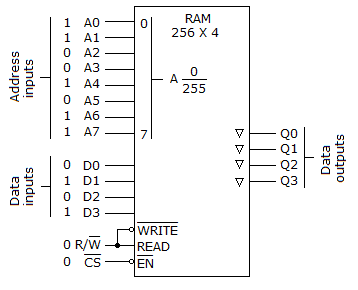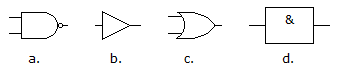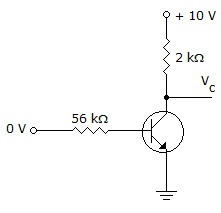# Online Digital Electronics Test - Digital Electronics Test - RandomLoading Test...

Instruction:

• This is a FREE online test. DO NOT pay money to anyone to attend this test.
• Total number of questions : 20.
• Time alloted : 30 minutes.
• Each question carry 1 mark, no negative marks.
• DO NOT refresh the page.
• All the best :-).

1.

Main computer memory is usually DRAM because of its high density and low cost; cache memory is usually SRAM because of its high speed.

A.
 TrueB.
 False2.

Static memory will maintain storage even if power is removed.

A.
 TrueB.
 False3.

The 8085A is a(n):

A.
 16-bit parallel CPUB.
 8-bit serial CPUC.
 8-bit parallel CPUD.
 none of the above4.

The inputs/outputs of an analog multiplexer/demultiplexer are:

A.
 bidirectionalB.
 unidirectionalC.
 even parityD.
 binary-coded decimal5.

A decoder can be used as a demultiplexer by ________.

A.
 tying all enable pins LOWB.
 tying all data-select lines LOWC.
 tying all data-select lines HIGHD.
 using the input lines for data selection and an enable line for data input6.

Which of the following expressions is in the sum-of-products form?

A.
 (A + B)(C + D)B.
 (AB)(CD)C.
 AB(CD)D.
 AB + CD7.

In VHDL, when we want to remember a value it must be stored in a VARIABLE.

A.
 TrueB.
 False8.

The base of the hexadecimal system is:

A.
 eight.B.
 sixteen.C.
 ten.D.
 two.9.

A.
 requires 16 adders.B.
 requires 4 adders.C.
 requires the carry-out of the less significant adder to be connected to the carry-in of the next significant adder.D.
 requires 4 adders and the connection of the carry out of the less significant adder to the carry-in of the next significant adder.10.

For the given circuit, which of the following is correct?A.
 The number 5 is being written to the memory at address location 203.B.
 The chip has not been enabled, since the EN terminal is 0; therefore, nothing will be written to the chip and the output is tri-stated.C.
 Decimal 10 is being written into memory location 211.D.
 The read/write line is LOW; therefore, decimal 5 is being stored at memory location 211.11.

Which of the symbols shown below represents an AND gate?A.
 aB.
 bC.
 cD.
 d12.

A ________ is a testing and troubleshooting tool that generates a short-duration pulse when manually activated, usually by depressing a push button.

A.
 cattle prodB.
 jimmy rodC.
 logic pulserD.
 bilateral switch13.

In the frequency counter, the pulse width of the enable signal is very critical for taking an accurate frequency measurement.

A.
 TrueB.
 False14.

Design costs for standard cell ASICs are ________ those for MPGAs.

A.
 lower thanB.
 about the same asC.
 higher thanD.
 none of the above15.

The field programmable logic array was the first ________ programmable logic device.

A.
 understandableB.
 logic arrayC.
 multifunctionD.
 nonmemory16.

What gives a GAL its flexibility?

A.
 Its speedB.
 Its reprogrammable EPROMC.
 Its large logic arraysD.
 Its programmable OLMCs17.

Why are the S and R inputs of a gated flip-flop said to be synchronous?

A.
 They must occur with the gate.B.
 They occur independent of the gate.18.

Master-slave J-K flip-flops are called pulse-triggered or level-triggered devices because input data is read during the entire time the clock pulse is at a LOW level.

A.
 TrueB.
 False19.

Is the transistor in the given figure considered ON or OFF?A.
 OFFB.
 ONC.
 Not enough information is provided.20.

The device that is an application of SOP logic is a multiplexer.

A.
 TrueB.
 False